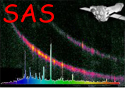XMM-Newton Science Analysis System

arfgen (arfgen-1.104) [xmmsas_20230412_1735-21.0.0]

## Main stage: Generate area

• Compute the source position in DET coordinates [xdet, ydet] from sourcex, sourcey: If the input coordinate system (form sourcecoords) is POS, convert sourcex, sourcey to EQPOS, using the attributes REFXCRVL and REFYCRVL from the spectrum as the reference point, then convert to DET using the spacecraft attitude at the start of the observation, taken from the OAL. If the coordinate system is EQPOS, convert to DET coordinates as above. If the parameter withsourcepos is false, then get the RA and DEC of the target from the attributes RA_PNT, DEC_PNT and convert to DET coordinates as with EQPOS above.

• Use xdet, ydet to determine the source position in TELCOORDs and compute mirror_area for that position.

• Compute sum of pixel values in the filtered detector map (detmap_sum).

• foreach detector pixel within the filtered detector map, across all CCDs

• Compute quantum efficiency curve (as a function of energy) for all selected patterns

• foreach mean energy value

• Compute the area for that pixel at that energy, in accordance to the following formula:(1)

The filter_transmission and quantum_efficiency values for each pixel are provided by the calibration data selected. The mirror area includes vignetting: for point sources this is calculated once at the source position, whereas for extended sources it is computed at the position of each detector map pixel.

• multiply product by the detector map pixel value.

• add result for pixel calculated above to running total at that energy

• end energy loop

• end pixel loop

• divide resultant area vector by detmap_sum

• correct area vector for encircled energy

• correct area vector for bad pixels/columns and CCD gaps

XMM-Newton SOC -- 2023-04-16